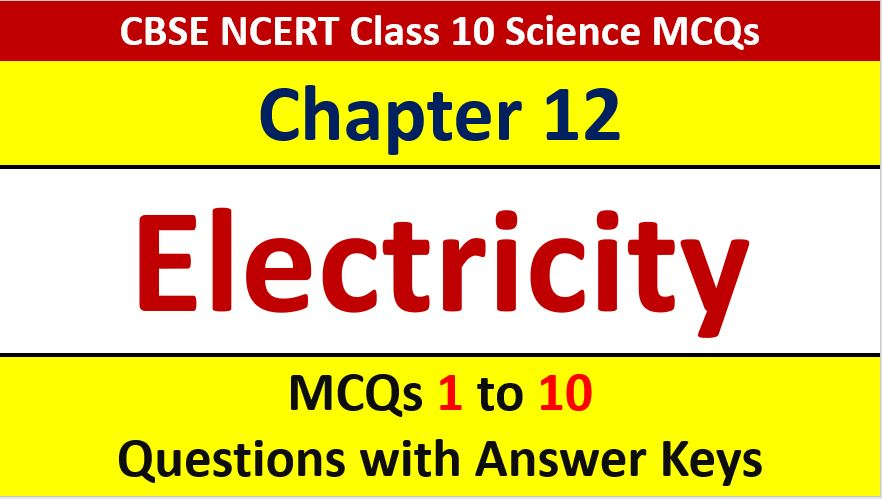## MCQ Questions for Class 10 Science Chapter 12 Electricity with Answer Keys and Solutions PDF

If you are preparing for CBSE Class 10 Board Exam Term 1 which will be an objective type questions paper, then you need to practice multiple choice questions of Class 10 Physics Chapterwise. In this article of AKVTutorials, you will get MCQ Questions for Class 10 Chapter 12 Electricity with Answer Keys.

MCQ Questions for Class 10 Science Chapter 12 Question No 1:

The rate of flow of charge is known as :

Option A : Electric potential

Option B : Electric conductance

Option C : Electric current

Option D : None of these

Option C : Electric current

MCQ Questions for Class 10 Science Chapter 12 Question No 2:

Which of the given is the SI Unit of Electric Current?

Option A : Ohm

Option B : Ampere

Option C : Volt

Option B : Ampere

MCQ Questions for Class 10 Science Chapter 12 Question No 3:

The instrument used for measuring electric current is :

Option A : Ammeter

Option B : Galvanometer

Option C : Voltmeter

Option D : Potentiometer

Option A : Ammeter

MCQ Questions for Class 10 Science Chapter 12 Question No 4:

The amount of work done in joules, when one unit electric charge moves from one point to another point in an electric circuit is called :

Option A : Electric Current

Option B : Electric Resistance

Option C : Electric Conductance

Option D : Potential Difference

Option D : Potential Difference

MCQ Questions for Class 10 Science Chapter 12 Question No 5:

The unit of potential difference is :

Option A : Volt

Option B : Ohm

Option C : Ampere

Option A : Volt

MCQ Questions for Class 10 Science Chapter 12 Question No 6:

The relation between potential difference (V) and current (I) is :

Option A : V ∝ I2

Option B : V ∝ 1/I

Option C : V∝ I

Option D : V ∝ I

Option D : V ∝ I

MCQ Questions for Class 10 Science Chapter 12 Question No 7:

The SI Unit of resistance is :

Option A : Newton

Option B : Ohm

Option C : Watt

Option D : Joule

Option B : Ohm

MCQ Questions for Class 10 Science Chapter 12 Question No 8:

A voltmeter is used to find potential difference in any electrical circuit which of the statement given below is true:

Option A : A voltmeter is a high resistance instrument and is connected in series circuit

Option B : A voltmeter is a low resistance instrument and is connected in series circuit

Option C : A voltmeter is a high resistance instrument and is connected in parallel circuit

Option D : A voltmeter is a low resistance instrument and is connected in parallel circuit

Option C : A voltmeter is a high resistance instrument and is connected in parallel circuit

MCQ Questions for Class 10 Science Chapter 12 Question No 9:

A battery of 12V is connected  in series with resistors of 0.2 ohm, 0.3 ohm, 0.4 ohm, 0.5 ohm, and 12 ohm. How much current would flow through the 0.3 ohm resistor?

Option A : 0.895 A

Option B : 1.11 A

Option C : 0.5 A

Option D : None of these

Option A : 0.895 A

MCQ Questions for Class 10 Science Chapter 12 Question No 10:

On which of the given resistance does not depend :

Option A : Length of conductor

Option B : Area of cross-section

Option C : Temperature

Option D : Density

Option D : Density

CBSE Class 10 Science Chapterwise MCQ
1 : Chemical Reactions & Equations MCQs
| 1 to 10 | 11 to 20
2 : Acids, Bases & Salts MCQs
| 1 to 10 | 11 to 20
3 : Metals & Non-Metals MCQs
| 1 to 10 | 11 to 20
4 : Carbon & Its Compound MCQs
| 1 to 10 | 11 to 20
5 : Periodic Classification of Elements MCQs
| 1 to 10 | 11 to 20
6 : Life Process MCQs
| 1 to 10 | 11 to 20
7 : Control & Coordination MCQs
| 1 to 10 | 11 to 20
8 : How Do Organism Reproduce MCQs
| 1 to 10 | 11 to 20
9 : Heredity & Evolution MCQs
| 1 to 10 | 11 to 20
10 : Light Reflection & Refraction MCQs
| 1 to 10 | 11 to 20
11 : Human Eye & Colorful World MCQs
| 1 to 10 | 11 to 20
12 : Electricity MCQs
| 1 to 10 | 11 to 20
13 : Magnetic Effect of Electric Current MCQs
| 1 to 10 | 11 to 20
14 : Source of Energy MCQs
| 1 to 10 | 11 to 20
15 : Our Environment MCQs
| 1 to 10 | 11 to 20
16 : Management of Natural Resources MCQs
| 1 to 10 | 11 to 20